# Multiplication Videos

These math videos demonstrate basic multiplication skills; each video solves a multiplication question. The videos concisely demonstrate the solution algorithm while highlighting key points.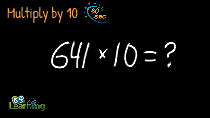Multiplying by 10 | Explained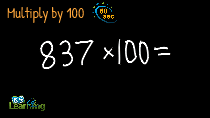Multiplying by 100 | Explained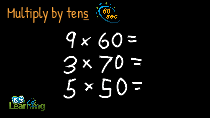Multiply by Tens | Explained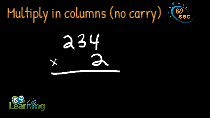Multiplying in Columns (No Carry) | Example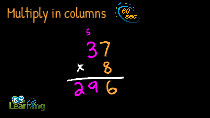Multiplying in Columns | Example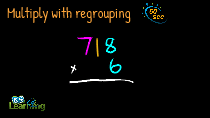Multiply with Regrouping | Example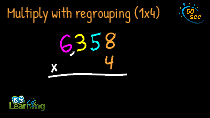Multiply with Regrouping (1x4 digits) | Example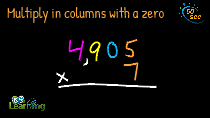Multiplying in columns | Example with Zero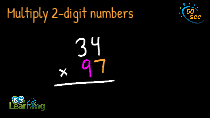Multiplying 2 Digit Numbers in Columns | Example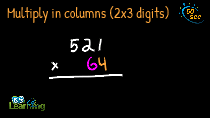Multiplying in columns (2x3 digits) | Example

What is K5?

K5 Learning offers free worksheets, flashcards and inexpensive workbooks for kids in kindergarten to grade 5. Become a member to access additional content and skip ads.